# Design Optimization of Induction Motor Using On-Line Improved Genetic Algorithms

Design Optimization of Induction Motor Using On-Line Improved Genetic Algorithms
Research Laboratory on the Electrical Engineering, University of M’Sila, 28000, Algeria

Signal & Systems Lab, Boumerdes University, Algeria

Corresponding Author Email:
salim.chakroun@univ-msila
Page:
19-25
|
DOI:
https://doi.org/10.18280/mmc_a.931-403
12 September 2020
|
Accepted:
3 December 2020
|
Published:
31 December 2020
| Citation

OPEN ACCESS

Abstract:

The main objective of this work is the application of a new architecture of genetic algorithms to the induction machine design in order to improve their performance. The latter is proposed by our research team based on modified crossing and mutation operators who have fixed values for conventional genetic algorithms. In addition, this version is characterized by a double loop and a random crossover. Firstly, to demonstrate the ability to locate the global optimum with this version algorithm a mathematical function was used. Then we approached the second phase which its application in real time to the induction motor optimized design problem. Knowing that, the machine is a highly coupled with multivariable system and constraints. Finally, the results obtained have been analyzed where we have found that satisfactory and can be declared that adaptation algorithm is effective in locating rapidly the region in which the global optimum exists in relation to the classical genetic algorithm.

Keywords:

induction motor design, optimization, genetic algorithm, improvements

1. Introduction

Nowadays, industry and infrastructure consume more than 31% of energy, and electric motors alone account for more than 60% of this consumption [1, 2]. In addition, the unavoidable disappearance of fossil reserves and the resulting inexorable cost increase compel manufacturers to make the best use of electrical energy. When making the decision to acquire a new machine, the investor should be led to consider the energy that will be consumed by this machine during its lifetime. Certainly, it is considered that since its acquisition until its dismantling, the purchase price represents 2 to 3% of the total cost , the rest being, mainly, the consumption of energy. This energy is necessarily attributed to the finished product and impacts the competitiveness of the company. Solutions exist to intelligently use available energy: Improve the efficiency of the machine, choose a running mode allowing to put in sleep the machines not much solicited, use the inverter, choose more energy-efficient movement strategies, use high efficiency motors [4, 5].

Since a simplicity of implementation, a small size, good performance and its excellent reliability. The induction motor is the most used motor in all industrial applications. Its only black spot is the reactive energy always consumed to magnetize the air gap [5, 6]. It is designed to operate at constant speed. However, it is increasingly associated with a drive which improves the flexibility of the machines. The use of a variable speed drive eliminates certain weaknesses of the induction motor: the starting current, the power factor and the voltage variations with inverter using the vector control .

In a related, nowadays the comparing induction motors efficiency is more complicated by the fact that there are several ways to measured them. Knowing that, the same motors evaluated according to different standards will be classified differently [8, 9]. The main standards are: Canadian CSA C390/M1985; American IEEE/ 112B; European IEC-34/2; JEC-37 Japanese. The Canadian Standards Association (CSA) takes into account the additional load losses which are indirectly measured. In addition the method used to measuring them is inspired by the IEEE. The CSA standard is, however more stringent than IEEE because it leaves little room for possible interpretation errors during yield. On the other hand, the IEEE standard calculates additional losses due to the load in an indirect way [1, 9]. It ranks second in terms of the caution of the results.

To improve the motor efficiency, several works are published in this area [1-3]. It is done by control so by maintenance or through design optimization which is the subject of this article. Despite the fact that, exist many optimization method and there are effort for improvement the old methods. So in this paper, we will focus on improving the efficiency of a closed rotor slot induction motor by proposing a solution during the design phase. The proposed approach is based on double loop modified genetic algorithms.

The results of the optimized design found are analyzed and validated or it can be declared that they are satisfactory.

2. Genetic Algorithm Optimization Techniques

The classical genetic algorithm (CGA) simulates biological evolutionary theories to solve optimization problems . They provide solutions by generating a set of chromosomes referred to as a generation. Each string has it is own fitness measure that reflects how well a creature can survive under the surrounding environment. The new generation of the strings is created through three major operations; selection, crossover and mutation, which provide a powerful global search mechanism which corresponds to 1st loop in Figure 1.

## 1.pngFigure 1. Proposed genetic algorithm diagram

Firstly, the selection is a process in which individual strings are copied into a mating pool according to their fitness values [10, 11]. Secondly, the crossover is a structured recombination operation. In the classical one-point crossover, a random position in a string is chosen and all characters to the right of this position are swapped. Finally, the mutation is an occasional random alteration of the value of a string position . As this version is linked to random criteria, the obtained optimization results differ from one execution to another. That is to say, there is a convergence towards a local optimum.

To remedy this problem, a new version has been proposed and applied in this paper. It is about modified genetic algorithms (MGA). So this improvement method always go to give a global solution for 80% of the test, by changing the probability of crossover Pc and mutation Pm operation in every one generation. The diagram of Figure 1 gives the clearest idea [1, 10]. The difference between the value of the current objective function and that of the previous generation is defined as "criteria 1" and maybe the generation number itself "criteria 2".

2.1 Operator function

In the proposed algorithm version, the second loop (SL) crossover operator Pc1 is calculated by the following function:

$P{{c}_{1}}=Pc+\frac{\left( 2*NGE+\left( NGE-1 \right) \right)}{{{N}^{k}}}$      (1)

where, Pc is the initial crossover value, NGE number of current generations for N number individuals and k is the bits number.

In the next, we will try to show the proposed modified principle for k =6, N=8. Not limited to a single crossover, but a second crossover to eliminate the bad individuals according to generation number.

 Parents P(1) 0 1 0 0 1 1 Parents P(2)  0 1 0 1 0 0 0 1 0 1 0 0 0 1 1 0 1 0 1 0 0 1 0 0 0 1 0 1 0 0 0 1 1 1 1 1 0 1 1 0 0 0 010100  Boy B(1)   010011  Boy B(2)  010010  011100  100100  010100 011000 011111 010100 Parents P'(1) 010011 010010 Parents P'(2) 011100 100100 010100 011100 011111 010010 Boy B'(1) 010100 010100 Boy B'(2) 011011 100111 010100 011100 011100 Selection CGA Crossover vector MGA (SL) Crossover vector

In addition, the improvement mutation operator in the second loop according to stop criterion 2 Pm1 is given according this formula:

$P{{m}_{1}}=Pm-\frac{\left( 2*NGE+\left( NGE-1 \right) \right)}{{{N}^{k}}}$     (2)

Such as Pm is the initial mutation value.

2.2 Test function

In order to validate the proposed algorithm, use a mathematical test function. This function knows has many local maximum as defined by:

\begin{align} & f({{x}_{1}},{{x}_{2}})=(4-2.1x_{{{1}^{{}}}}^{2}\cdot x_{1}^{4/3})x_{1}^{2} \\ & +{{x}_{1}}\cdot \text{ }{{\text{x}}_{\text{2}}}+\text{(-4}+\text{4}{{\text{x}}_{\text{2}}}{{^{2}}^{{}}})x_{2}^{2} \\\end{align}       (3)

where, the search space is such that: [x1,x2]∈{[-2,-2] and [2,2]}.

Taking into account the modifications listed above, a program based on genetic algorithms has been developed. To check the validity of this program, a series of five consecutive executions was used and we obtained the following results:

## 2.pngFigure 2. Best fitness function evolution with CGA

## 3.pngFigure 3. Error evolution for CGA algorithm

We can see in Figure 2 and 3 that for each execution the algorithm converges to a local optimum and the error is significant before becoming zero after 350 generations. That is to say that for each execution we have a maximum, this is justified by the stochastic criterion linked to classical genetic algorithms.

On the other hand, in the case of the modified genetic algorithms Figure 4, one has the same results for the five executions after the 250 generations among the 400 considered witch illustrated in Table 1. This is proof of the proposed algorithm despite the major drawback which is the important execution time.

## 4.pngFigure 4. Best fitness function evolution with MGA

In addition we have in Figure 5 the error tends to a very low value and stable after a great initial value. Which makes us go deeper, that is applying this algorithm to the complex and restrictive problem.

## 5.pngFigure 5. Error evolution for MGA algorithm

Through the global results witch grouped in table 1, we can see that we have a vary results from one execution to another. On the other hand for the case of modified genetic algorithms, the results seem to be the same with a very small and negligible difference.

Table 1. Results for test function

 Execution     Number CGA Results MGA Results x1 x2 Max f(x1, x2) x1 x2 Max f(x1, x2) 1 -0.8571 1.2380 0.7665 2 -0.0317 1.9979 2 2 0.6031 1.4664 2 -0.0952 1.9820 3 2 1.4285 0.6577 2 -0.0952 1.9820 4 2 1.6190 0.5522 -0.8571 0.0317 1.9397 5 2 0.0317 1.9979 -0.9841 0.0952 1.9812

So, this result are encouraging and paves the way for reverting to the application of genetic algorithms in optimization problem generally and precisely in the design optimized system field. The latter is the focus of our paper.

3. Induction Motor Design Optimization

In order to design of electrical machines, Marcel JUFER and Jean-Claude propose a dimensioning of the stator iron then the rotor calculation method . A second pedagogical method used at the polytechnic school from Montreal for didactic. The third procedure combines computation and computer-assisted design of an induction machine, and takes different stages of calculation in chronological order. This approach is based on the design principle of G. Kouskoff and Liwschitz. Except that we impose the geometric data of an already existing industrial machine, as constraints to which we must submit. The numerical results of the calculation are compared with those given by the classical test method and then processed by a simulated dynamic analysis of the behaviors of the machine in order to develop the correlation and concordance of these design results with those delivered by the manufacturer [12, 13]. Finally there is another method named Liwschitz method, based on the flowchart presented in Figure 6.

The preceding method is coupled to the prescribed genetic algorithm version and is applied in our contribution on a closed rotor induction machine with: 5kW, 4 poles, 1440trs/min, 380V, Δ coupled. In addition to that, this machine has a specificity concerning the stator slot depth.

## 6.png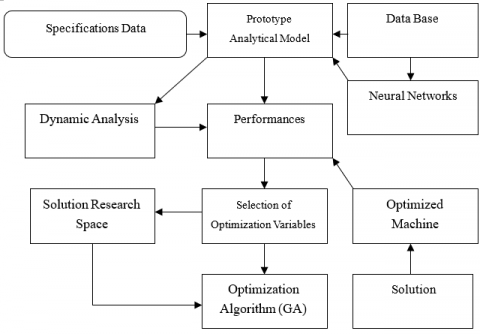Figure 6. Proposed and apply induction motor design optimization flowchart

Using empirical equations and a database to determine the main dimensions of this machine [1, 2], a design program has been developed by our group [14, 15].

Through the obtained results, we define the boundary limits and search space of the five optimization variables [1, 16]. These variables are geometric, magnetic and general with a constraint such as the starting torque which does not exceed 90Nm. In addition to this, the motor efficiency (Eff) is the fitness function. For the consecutive five executions, we got the results grouped in the following.

## 7.png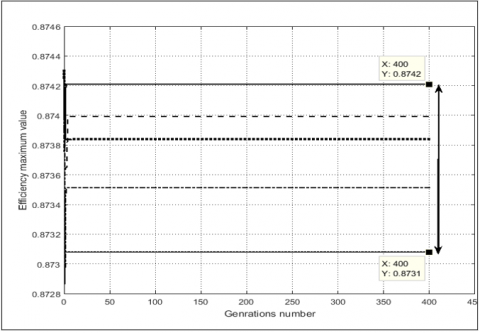Figure 7. Best fitness function evolution with CGA

## 8.png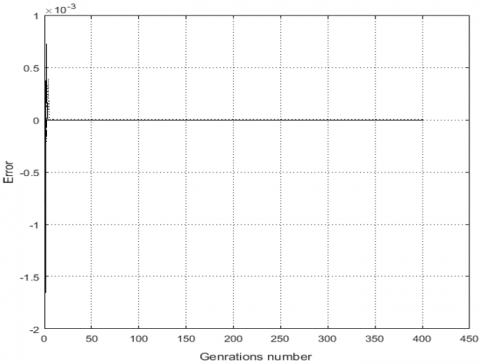Figure 8. Error evolution for CGA algorithm

## 9.png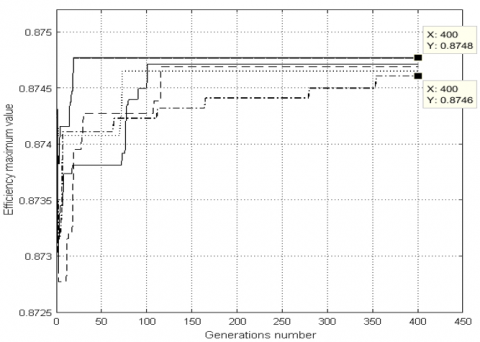Figure 9. Results for design problem with MGA

## 10.png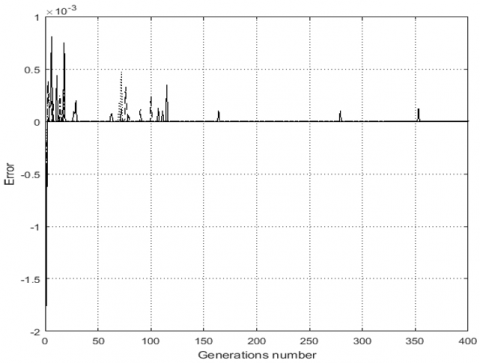Figure 10. Error evolution for MGA

## 11.pngFigure 11. Crossover operator improvement in MGA algorithm

## 12.pngFigure 12. Mutation operator evolution in MGA algorithm

Through Figure 9, we note for the proposed version results that 350 generations are sufficient for the algorithm to converge, unlike for the classic version Figure 7 which requires a larger number. On the other hand the error is minimal it always remains lower by 10-3 and Pc value decreases to 0.69 depending on the stopped criteria. With respect in Figure 12 Pm reaches a maximum value of 0.02, it remains constant until 400 generations.

Finally, we can declare that the developed algorithm is still effective for complex and multivariable problems. But the only downside is the simulation time, which can be a bit long.

Since, in this paper, the finesse function is the machine efficiency. It is 0.874 compared to the existing machine (CM) Effi = 0.85. This improvement is accompanied by a low Pc and a higher Pm. Indeed, all the results found are shown in Table 2. We note that the opening rotor notch is a key parameter to improve the induction machines efficiency because it is always in the lower limit as the interior diameter for all time, opposite for the air gap flux density [1, 16].

The obtained results by the combination between design program and the proposed optimization algorithm are used in another program based on the equivalent diagram method [1, 12]. On which, the usual characteristics of the studied machine can be plotted in static mode. This is illustrated by the next figures.

We can see the efficiency versus useful power for each machine on Figure 13. This figure shows that in the vicinity of the nominal point (5kW) the efficiency of the machine optimized by the modified genetic algorithms (MGA) is better compared to the design optimized by conventional genetic algorithms (CGA) as well as the machine exists.

In addition, Figure 14 shows the characteristic of the stator phase currents for each machine. However, the difference is notable this for the maximum useful power and for the starting current or the difference reached 3A, this is encouraging for future application and for more complicated systems.

## 13.png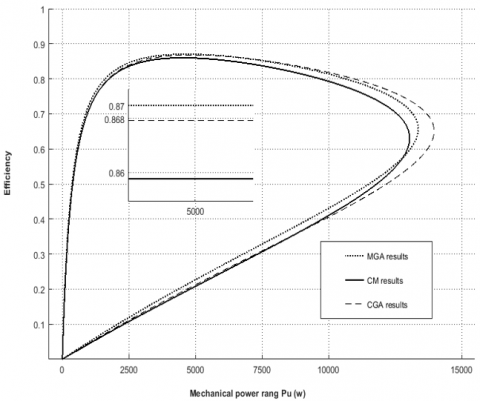Figure 13. Efficiency versus mechanical power

Via the Park model (d, q) in order to modelling this motor then check the capacity of the optimized machine under dynamic conditions. We apply to the machines various torque: Nominal torque (Tn), starting torque (Ts) at t=1s, the maximum torque (Tmx) for t= 2s and the torque greater than the maximum torque (Tbg) at t=3s.

Table 2. Results of induction motor design optimization

 Execution Number CGA Results Initial operators: Pc =0.85, Pm=0.01 Variable vector X and limit Pc Pm Eff(X) x1 [m] Stator internal diameter [149e-3:153e-3] x2 Geometric rapport [ 1.1: 1.3] x3 [m] Stator notch depth [17e-3: 21e-3] x4 [T] Induction in the gap [0.59: 0.63] x5 [m] Rotor notch opening [0.5e-3: 0.9e-3] 1 0.1506 1.2968 0.0175 0.6236 0.000626 0.85 0.01 0.873 2 0.1521 1.1984 0.0197 0.6249 0.000849 0.85 0.01 0.873 3 0.1509 1.2619 0.0175 0.6090 0.000690 0.85 0.01 0.873 4 0.1530 1.1920 0.0211 0.6299 0.000900 0.85 0.01 0.872 5 0.1505 1.2619 0.0170 0.6261 0.000658 0.85 0.01 0.873 MGA Results Initial operators: Pc =0.85,   Pm=0.01 Variable Vector X final Pc final Pm Eff(X) x1 x2 x3 x4 x5 1 0.15220 1.2492 0.01712 0.61476 0.000607 0.741 0.2 0.874 2 0.15173 1.1317 0.01764 0.59102 0.000510 0.752 0.2 0.874 3 0.15109 1.1571 0.01715 0.61032 0.000523 0.752 0.2 0.874 4 0.15065 1.1222 0.02080 0.62809 0.000569 0.752 0.2 0.874 5 0.15013 1.3144 0.01715 0.62301 0.000531 0.696 0.2 0.874

## 14.pngFigure 14. Stator phase current versus mechanical power

The results of this test are the different characteristics of the three machines: the speed, the electric current, the electromagnetic torque and all the results are grouped in figures below.

## 151.pnga- Electromagnetic torque versus time

## 152.pngb- Rotor speed versus time

## 153.png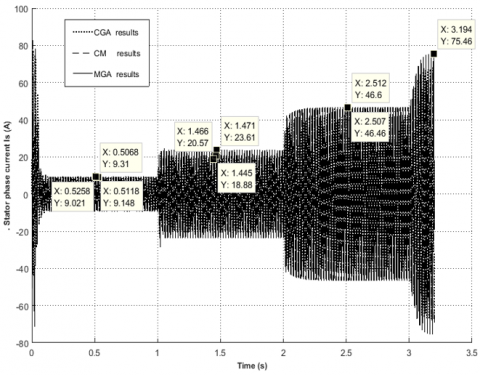c- Stator phase current versus time

Figure 15. Results of dynamical mode

We note that the machine optimized by the new version (MGA PC Variable) has better dynamical performance from the point of view overload (126.6Nm) and start-up capacity (84.14Nm). These properties accompanied by a low speed variation and a less call current opposite to the machine optimized by the classical genetic algorithms (CGA PC fixe) and the classical machine (MC), this is be fond of second validation method.

Then again, it can be observe that the speed of the machine optimized by MGA is less susceptible to the load deviation compared to the other two machines. This specificity is accompanied by a better starting capacity and overloading this is important for industrial applications.

4. Conclusion

In this paper we tried to develop and apply in real time a version of genetic algorithms. Certainly, according to a stopping criterion a second loop with a second crossover has been proposed. The latter is random based on variable crossover and mutation operators. To test the ability to locate the global optimum by this version algorithm, an application to the mathematical function as used then optimizing design problem of an induction machine has been proposed. The found results either for static or dynamic analysis is satisfactory and open up perspectives towards other more complicated applications.

References

 Chekroun, S., Abdelhadi, B., Benoudjit, A. (2014). A New approach design optimizer of induction motor using particle swarm algorithm. Modelling, Measurement and Control A, 87(2): 89-108.

 Zeghba, O., Chakroune, S., Belhamdi, S. (2018). Multi objective design of high efficiency induction motor using an analytical method's. AMSE. Journals, Modelling. A, 91(4): 202-211.

 Benoudjit, A., Abdelhadi, B., Said, N.N., Idir, S. (2002). High efficiency induction motors-impact on energy saving potential in Algeria. In Conference on Electrical Engineering, CEE02, 1-6.

 Boglietti, A., Cavagnino, A., Ferraris, L., Lazzari, M., Luparia, G. (2005). No tooling cost process for induction motors energy efficiency improvements. IEEE transactions on industry applications, 41(3): 808-816. https://doi.org/10.1109/TIA.2005.847309

 Salana, K.S., Singh, T., Singh, N. (2010). Saving energy using energy efficient motors: A case study. https://doi.org/10.1049/cp.2010.0004

 Dessus, B. (1987). Maîtrise de l'énergie et coût de mise à disposition d'un service. Revue de l'énergie, 392: 235-244.

 Angioletti, R., Despretz, H. (2004). Maîtrise de l'énergie dans les bâtiments-définitions. usages. consommations. Techniques des ingénieurs.(cité pages 31, 32, 34, 36, 43, 173 et 175.).

 Boglietti, A., Lazzari, M., Pastorelli, M. (2000). A simplified method for the iron losses prediction in soft magnetic materials with arbitrary voltage supply. In Conference Record of the 2000 IEEE Industry Applications Conference. Thirty-Fifth IAS Annual Meeting and World Conference on Industrial Applications of Electrical Energy (Cat. No. 00CH37129), 1: 269-276. https://doi.org/10.1109/IAS.2000.881117

 Singh, N., Brar, N., Dhingra, A. (2015). A case study of energy saving using energy efficient motors in a process plant. International Journal of Engineering and Advanced Technology, 4(5): 90-92.

 Man, K.F., Tang, K.S., Kwong, S. (1996). Genetic algorithms: concepts and applications [in engineering design]. IEEE transactions on Industrial Electronics, 43(5): 519-534. https://doi.org/10.1109/41.538609

 Chekroun, S., Abdelhadi, B., Benoudjit, A. (2008). Design optimization of induction motor efficiency by genetic algorithms. AMSE. Journal, 14-29.

 Boglietti, A., Cavagnino, A., Lazzari, M., Pastorelli, M. (2001). Two simplified methods for the iron losses prediction in soft magnetic materials supplied by PWM inverter. In IEMDC 2001. IEEE International Electric Machines and Drives Conference (Cat. No. 01EX485), pp. 391-395.

 Boglietli, A., Cavagnino, A., Lazzari, M., Pastorelli, A. (2003). Induction motor efficiency measurements in accordance to IEEE 112-B, IEC 34-2 and JEC 37 international standards. In IEEE International Electric Machines and Drives Conference, 2003. IEMDC'03., 3: 1599-1605. https://doi.org/10.1109/IEMDC.2003.1210664

 Çunkaş, M., Akkaya, R. (2006). Design optimization of induction motor by genetic algorithm and comparison with existing motor. Mathematical and Computational Applications, 11(3): 193-203. https://doi.org/10.3390/mca11020193

 Abdelhadi, B., Benoudjit, A., Nait Said, N. (2004). Identification of induction machine parameters using a new adaptive genetic algorithm. Electric Power Components and Systems, 32(8): 767-784. https://doi.org/10.1080/15325000490466645

 Faiz, J., Sharifian, M.B.B. (2006). Optimal design of an induction motor for an electric vehicle. European Transactions on Electrical Power, 16(1): 15-33. https://doi.org/10.1002/etep.62